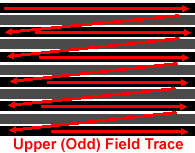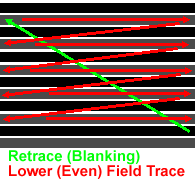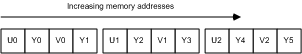# yuv rgb

2013/09/18 11:14

RGB介绍

YUV介绍

RGB转YUV

YUV转RGB(图1 上场扫描)(图2 下场扫描)

RGB介绍：

YUV介绍：

YUV是被欧洲电视系统所采用的一种颜色编码方法，我国广播电视也普遍采用这类方法。其中“Y”表示明亮度（Luminance或Luma），也就是灰阶值；而“U”和“V”表示的则是色度（Chrominance或Chroma）。彩色电视采用YUV空间正是为了用亮度信号Y解决彩色电视机与黑白电视机的兼容问题，使黑白电视机也能接收彩色电视信号。

BMP图像颜色信息的保存顺序是由左到右，由下往上，您可以执行一下附带程序的 (功能菜单->读取RGB) 看到图像的读取和显示过程。代码首先依次显示奇数行像素，如(1，3，5，7，9….行)，完成后再依次显示偶数行像素，代码实现如下：

01. // 隔行显示BMP
03. {
05. CDC *pDC = GetDC();
06.
07. CRect rect;
08. CBrush brush(RGB(128,128,128));
09. GetClientRect(&rect);
10. pDC->FillRect(&rect, &brush);
11.
14.
15. char strFileName[MAX_PATH]="720bmp.bmp";
16. CFile* f;
17. f = new CFile();
19. f->SeekToBegin();
22.
23. // 分配图片像素内存
24. RGBTRIPLE *rgb;
25. rgb = new RGBTRIPLE[bmih.biWidth*bmih.biHeight];
26.
27. f->SeekToBegin();
28. f->Seek(54,CFile::begin); // BMP 54个字节之后的是像素数据
29. f->Read(rgb, bmih.biWidth * bmih.biHeight * 3);   // 这里只读24位RGB(r,g,b)图像
30.
31. // 显示上场 (奇数行组成的奇数场)
32. for (int i = 0; i < bmih.biHeight; i++) {
33. for (int j = 0; j < bmih.biWidth; j++) {
34. if(!(i%2))
35. pDC->SetPixel(j, bmih.biHeight-i,
36. RGB(rgb[i*bmih.biWidth+j].rgbtRed,
37. rgb[i*bmih.biWidth+j].rgbtGreen,rgb[i*bmih.biWidth+j].rgbtBlue));
38. for (int k=0; k< 1000; k++) ;  //延时
39. }
40. }
41.
42. Sleep(500);
43.
44. // 显示下场 (偶数行组成的偶数场)
45. for (int i_ = 0; i_< bmih.biHeight; i_++) {
46. for (int j_ = 0; j_< ;bmih.biWidth; j_++) {
47. if(i_%2)
48. pDC->SetPixel(j_, bmih.biHeight-i_,
49. RGB(rgb[i_*bmih.biWidth+j_].rgbtRed,
50. rgb[i_*bmih.biWidth+j_].rgbtGreen,
51. rgb[i_*bmih.biWidth+j_].rgbtBlue));
52. for (int k=0; k< 1000; k++) ;  //延时
53. }
54. }
55.
56. // 显示24位BMP信息
57. LONG dwWidth = bmih.biWidth;
58. LONG dwHeight = bmih.biHeight;
59. WORD wBitCount = bmih.biBitCount;
60. char buffer;
61. sprintf(buffer,"图像宽为：%ld 高为：%ld 像数位数：%d", dwWidth, dwHeight, wBitCount);
62. MessageBox(buffer, "每个像素的位数", MB_OK | MB_ICONINFORMATION);
63.
64. f->Close();
65. delete f;
66. delete rgb;
67. }

RGB转YUV(图3 UYVY字节顺序)

[U0，Y0，U1，Y1] [U1，Y2，V1，Y3] [U2，Y4，V2，Y5] ……

RGB转UYVY公式如下：

Y = 0.257R′ + 0.504G′ + 0.098B′ + 16

Cb = -0.148R′ - 0.291G′ + 0.439B′ + 128

Cr = 0.439R′ - 0.368G′ - 0.071B′ + 128

01. // RGB转换为YUV
02. void CRGB2YUVView::RGB2YUV(byte *pRGB, byte *pYUV)
03. {
04. byte r,g,b;
05. r = *pRGB; pRGB++;
06. g = *pRGB; pRGB++;
07. b = *pRGB;
08.
09. *pYUV = static_cast< byte >(0.257*r + 0.504*g + 0.098*b + 16);    pYUV++;   // y
10. *pYUV = static_cast< byte >(-0.148*r - 0.291*g + 0.439*b + 128);  pYUV++;   // u
11. *pYUV = static_cast< byte >(0.439*r - 0.368*g - 0.071*b + 128);             // v
12. }

001. // 转换RGB
002. void CRGB2YUVView::OnConvertPAL()
003. {
004. CDC *pDC = GetDC();
005. CRect rect;
006. CBrush brush(RGB(128,128,128));
007. GetClientRect(&rect);
008. pDC->FillRect(&rect, &brush);
009.
010. // PAL 720x576 : 中国的电视标准为PAL制
011. int CurrentXRes = 720;
012. int CurrentYRes = 576;
013. int size        = CurrentXRes * CurrentYRes;
014.
015. // 分配内存
016. byte *Video_Field0 = (byte*)malloc(CurrentXRes*CurrentYRes);
017. byte *Video_Field1 = (byte*)malloc(CurrentXRes*CurrentYRes);
018.
019. // 保存内存指针
020. byte *Video_Field0_ = Video_Field0;
021. byte *Video_Field1_ = Video_Field1;
022.
023. byte yuv_y0, yuv_u0, yuv_v0, yuv_v1;  // {y0, u0, v0, v1};
024. byte bufRGB;  // 临时保存{R,G,B}
025. byte bufYUV;  // 临时保存{Y,U,V}
026.
027. // 初始化数组空间
028. ZeroMemory(bufRGB, sizeof(byte)*3);
029. ZeroMemory(bufYUV, sizeof(byte)*3);
030.
031. // 初始化内存
032. ZeroMemory(Video_Field0, CurrentXRes*CurrentYRes);
033. ZeroMemory(Video_Field1, CurrentXRes*CurrentYRes);
034.
035. // BMP 位图操作
038.
039. char strFileName[MAX_PATH]="720bmp.bmp";
040. CFile* f;
041. f = new CFile();
043. f->SeekToBegin();
046.
047. // 分配图片像素内存
048. RGBTRIPLE *rgb;
049. rgb = new RGBTRIPLE[bmih.biWidth*bmih.biHeight];
050.
051. f->SeekToBegin();
052. f->Seek(54,CFile::begin);  // BMP 54个字节之后的是位像素数据
053. f->Read(rgb, bmih.biWidth * bmih.biHeight * 3);   // 这里只读24位RGB(r,g,b)图像
054.
055. // 上场  (1,3,5,7...行)
056. for (int i = bmih.biHeight-1; i>=0; i--) {
057. for (int j = 0; j< bmih.biWidth; j++) {
058. if(!(i%2)==0)
059. {
060. bufRGB = rgb[i*bmih.biWidth+j].rgbtRed;   // R
061. bufRGB = rgb[i*bmih.biWidth+j].rgbtGreen; // G
062. bufRGB = rgb[i*bmih.biWidth+j].rgbtBlue;  // B
063.
064. // RGB转换为YUV
065. RGB2YUV(bufRGB,bufYUV);
066. yuv_y0 = bufYUV;   // y
067. yuv_u0 = bufYUV;   // u
068. yuv_v0 = bufYUV;   // v
069.
070. for (int k=0; k< 1000; k++) ;  //延时
071. // 视图中显示
072. pDC->SetPixel(j, (bmih.biHeight-1)-i, RGB(bufRGB, bufRGB, bufRGB));
073.
074. // UYVY标准  [U0 Y0 V0 Y1] [U1 Y2 V1 Y3] [U2 Y4 V2 Y5]
075. // 每像素点两个字节，[内]为四个字节
076. if ((j%2)==0)
077. {
078. *Video_Field0 = yuv_u0;
079. Video_Field0++;
080. yuv_v1 = yuv_v0;   // v保存起来供下一字节使用
081. }
082. else
083. {
084. *Video_Field0 = yuv_v1;
085. Video_Field0++;
086. }
087. *Video_Field0 = yuv_y0;
088. Video_Field0++;
089. }// end if i%2
090. }
091. }
092.
093. // 下场 (2,4,6,8...行)
094. for (int i_ = bmih.biHeight-1; i_>=0; i_--) {
095. for (int j_ = 0; j_< bmih.biWidth; j_++) {
096. if((i_%2)==0)
097. {
098. bufRGB = rgb[i_*bmih.biWidth+j_].rgbtRed;   //   R
099. bufRGB = rgb[i_*bmih.biWidth+j_].rgbtGreen; // G
100. bufRGB = rgb[i_*bmih.biWidth+j_].rgbtBlue;  // B
101.
102. // RGB转换为YUV
103. RGB2YUV(bufRGB,bufYUV);
104. yuv_y0 = bufYUV;   // y
105. yuv_u0 = bufYUV;   // u
106. yuv_v0 = bufYUV;   // v
107.
108. for (int k=0; k< 1000; k++) ;  //延时
109. // 视图中显示
110. pDC->SetPixel(j_, (bmih.biHeight-1)-i_, RGB(bufRGB, bufRGB, bufRGB));
111.
112. // UYVY标准  [U0 Y0 V0 Y1] [U1 Y2 V1 Y3] [U2 Y4 V2 Y5]
113. // 每像素点两个字节，[内]为四个字节
114. if ((j_%2)==0)
115. {
116. *Video_Field1 = yuv_u0;
117. Video_Field1++;
118. yuv_v1 = yuv_v0;   // v保存起来供下一字节使用
119. }
120. else
121. {
122. *Video_Field1 = yuv_v1;
123. Video_Field1++;
124. }
125. *Video_Field1 = yuv_y0;
126. Video_Field1++;
127. }
128. }
129. }
130.
131. // 关闭BMP位图文件
132. f->Close();
133. WriteYUV(Video_Field0_, Video_Field1_, size);
134.
135. // 释放内存
136. free( Video_Field0_ );
137. free( Video_Field1_ );
138. delete f;
139. delete rgb;
140. }

YUV转RGB

12个字节的UYVY排列方式：

[U0 Y0 V0 Y1] [U1 Y2 V1 Y3] [U2 Y4 V2 Y5]

[Y0 U0 V0] [Y1 U1 V1] [Y2 U2 V2] [Y3 U3 V3] [Y4 U4 V4] [Y5 U5 V5]

1. //  反变换公式
2. R= 1.0Y + 0 +1.402(V-128)
3. G= 1.0Y - 0.34413 (U-128)-0.71414(V-128)
4. B= 1.0Y + 1.772 (U-128)+0

001. void CRGB2YUVView::YUV2RGB(byte *pRGB, byte *pYUV)
002. {
003. byte y, u, v;
004. y = *pYUV; pYUV++;
005. u = *pYUV; pYUV++;
006. v = *pYUV;
007.
008. *pRGB = static_cast< byte >(1.0*y + 8 + 1.402*(v-128));    pRGB++;                 // r
009. *pRGB = static_cast< byte >(1.0*y - 0.34413*(u-128) - 0.71414*(v-128));  pRGB++;   // g
010. *pRGB = static_cast< byte >(1.0*y + 1.772*(u-128) + 0);                            // b
011. }
012.
013. // 读取PAL文件转换为RGB并显示
015. {
017. CDC *pDC = GetDC();
018. CRect rect;
019. CBrush brush(RGB(128,128,128));
020. GetClientRect(&rect);
021. pDC->FillRect(&rect, &brush);
022.
023. // PAL 720x576 : 中国的电视标准为PAL制
024. int CurrentXRes = 720;
025. int CurrentYRes = 576;
026. int size        = CurrentXRes * CurrentYRes;
027.
028. // 分配内存
029. byte *Video_Field0 = (byte*)malloc(CurrentXRes*CurrentYRes);
030. byte *Video_Field1 = (byte*)malloc(CurrentXRes*CurrentYRes);
031.
032. // 保存内存指针
033. byte *Video_Field0_ = Video_Field0;
034. byte *Video_Field1_ = Video_Field1;
035.
036. // 初始化内存
037. ZeroMemory(Video_Field0, CurrentXRes*CurrentYRes);
038. ZeroMemory(Video_Field1, CurrentXRes*CurrentYRes);
039.
040. byte yuv_y0, yuv_u0, yuv_v0; // yuv_v1;  // {y0, u0, v0, v1};
041. byte r, g, b;
042. byte bufRGB;  // 临时保存{R,G,B}
043. byte bufYUV;  // 临时保存{Y,U,V}
044.
045. // 初始化数组空间
046. memset(bufRGB,0, sizeof(byte)*3);
047. memset(bufYUV,0, sizeof(byte)*3);
048.
049. char strFileName[MAX_PATH]="720bmp.pal";
050.
051. // 分配图片像素内存
052. RGBTRIPLE *rgb;
053. rgb = new RGBTRIPLE[CurrentXRes*CurrentYRes];
054.
055. memset(rgb,0, sizeof(RGBTRIPLE)*CurrentXRes*CurrentYRes); // 初始化内存空间
056.
057. CFile* f;
058. f = new CFile();
060. f->SeekToBegin();
063.
064. // 上场  (1,3,5,7...行)
065. for ( int i = CurrentYRes-1; i>=0; i--) {
066. for ( int j = 0; j< CurrentXRes; j++) {
067. if(!(i%2)==0)
068. {
069. // UYVY标准  [U0 Y0 V0 Y1] [U1 Y2 V1 Y3] [U2 Y4 V2 Y5]
070. // 每像素点两个字节，[内]为四个字节
071. if ((j%2)==0)
072. {
073. yuv_u0 = *Video_Field0;
074. Video_Field0++;
075. }
076. else
077. {
078. yuv_v0 = *Video_Field0;
079. Video_Field0++;
080. }
081. yuv_y0 = *Video_Field0;
082. Video_Field0++;
083.
084. bufYUV = yuv_y0;  // Y
085. bufYUV = yuv_u0;  // U
086. bufYUV = yuv_v0;  // V
087.
088. // RGB转换为YUV
089. YUV2RGB(bufRGB,bufYUV);
090. r = bufRGB;   // y
091. g = bufRGB;   // u
092. b = bufRGB;   // v
093. if (r>255) r=255; if (r< 0) r=0;
094. if (g>255) g=255; if (g< 0) g=0;
095. if (b>255) b=255; if (b< 0) b=0;
096.
097. for (int k=0; k< 1000; k++) ;  //延时
098. // 视图中显示
099. pDC->SetPixel(j, CurrentYRes-1-i, RGB(r, g, b));
100.
101. }// end if i%2
102. }
103. }
104.
105. // 下场 (2,4,6,8...行)
106. for ( int i_ = CurrentYRes-1; i_>=0; i_--) {
107. for ( int j_ = 0; j_< CurrentXRes; j_++) {
108. if((i_%2)==0)
109. {
110. // UYVY标准  [U0 Y0 V0 Y1] [U1 Y2 V1 Y3] [U2 Y4 V2 Y5]
111. // 每像素点两个字节，[内]为四个字节
112. if ((j_%2)==0)
113. {
114. yuv_u0 = *Video_Field1;
115. Video_Field1++;
116. }
117. else
118. {
119. yuv_v0 = *Video_Field1;
120. Video_Field1++;
121. }
122. yuv_y0 = *Video_Field1;
123. Video_Field1++;
124.
125. bufYUV = yuv_y0;  // Y
126. bufYUV = yuv_u0;  // U
127. bufYUV = yuv_v0;  // V
128.
129. // RGB转换为YUV
130. YUV2RGB(bufRGB,bufYUV);
131. r = bufRGB;   // y
132. g = bufRGB;   // u
133. b = bufRGB;   // v
134. if (r>255) r=255; if (r< 0) r=0;
135. if (g>255) g=255; if (g< 0) g=0;
136. if (b>255) b=255; if (b< 0) b=0;
137.
138. for (int k=0; k< 1000; k++) ;  //延时
139. // 视图中显示
140. pDC->SetPixel(j_, CurrentYRes-1-i_, RGB(r, g, b));
141. }
142. }
143. }
144.
145. // 提示完成
146. char buffer;
147. sprintf(buffer,"完成读取PAL文件：%s ", strFileName);
148. MessageBox(buffer, "提示信息", MB_OK | MB_ICONINFORMATION);
149.
150. // 关闭PAL电视场文件
151. f->Close();
152.
153. // 释放内存
154. free( Video_Field0_ );
155. free( Video_Field1_ );
156. delete f;
157. delete rgb;
158. }

Video Rendering with 8-Bit YUV Formats

….

QQ:15385155 MAIL: liyingjiang@21cn.com

0
0 收藏

### 作者的其它热门文章0 评论
0 收藏
0